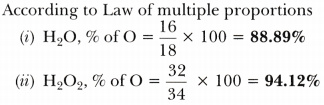# Calculate the mass percentage of oxygen present in the following compounds and state the law of chemical combination associated. Given, H = 1, O = 16. Water (H20) and Hydrogen peroxide (H202)

Calculate the mass percentage of oxygen present in the following compounds and state the law of chemical combination associated. Given, H = 1, O = 16.

• Water (H20) and
• Hydrogen peroxide (H202)